﻿ 基于FPGA的水声信号数字下变频的实现
 舰船科学技术2016, Vol. 38Issue (8): 90-94PDF

The implementation of digtal down conversion of underwater acoustic signal with FPGA
TANG Wei-jie
The 715 Research Institute of CSIC, Hangzhou 310012, China
Abstract: With the development of sonar signal process, more and more software or hardware resources are required. It can greatly reduced just use Digtal Down Conversion(DDC). In the paper, simulation was done to study the DDC of Underwater Acoustic Signal in Matlab, and results were given and analyzed. Then the digtal signal processing was programmed by Verilog in FPGA. Variables and results were checked in Signaltap Ⅱ and analyzed in Matlab. This technology have practical value to signal processing of Sonarand have been sucessed applied in the Sonar system.
Key words: sonar     underwater acoustic signal     filter     down sample     FPGA
0 引  言

1 基本原理

1.1 数字下变频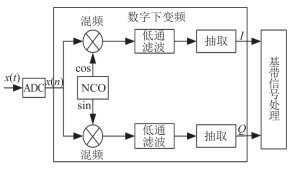图 1 DDC的原理框图 Fig. 1 Schematic diagram of DDC
1.2 数控振荡

 $s(n) = \sin (2\pi {f_0} \cdot /{f_s}),(n = 0,1,2, \cdot \cdot \cdot )。$

DDC以${\rm{1/}}{f_s}$的采样时钟周期每输入一个样本数据，NCO的相位累加器相应增加一个 $2{\rm{\pi}}{f_0}\cdot/{f_s}$的增量，以累加后的相位值作为地址，读出该地址上的样本作为正弦值，以此循环。此查找表预先存入ROM中，若相位位数N越大，要求的分辨率越高，其存储的ROM空间越大。如果要提高分辨率，往往要消耗大量的存储资源，因此可能受限于系统的存储空间。

1.3 混频器

 $x(t)=a(t)\cos[{\omega_0}t+\phi(t)]。$

 $s(t)=a(t){e^{j\phi(t)}}{e^{j{\omega_0}t}}。$

$a(t)$$\phi(t)分别为信号的幅度调制分量和相位调制分量；{\omega_0}为信号的载频。因此，s(t)$${e^{-j{w_0}t}}$相乘，即有：

1.4 FIR低通滤波

 $y(n)=\sum\limits_{k=-\infty}^\infty{h(k)\cdot(n-k)}，$

 $H({e^{j\omega }}) = \sum\limits_{k = 0}^{N - 1} {h(k) \cdot {e^{ - j\omega k}}}。$

 $h(n) = \frac{1}{{2\pi }}\int_{ - \pi }^\pi {H({e^{j\omega }}){e^{j\omega n}}} {\rm{d}}\omega 。$

1.5 多速率信号抽取

 $x(m)=x(mN)。$

2 Matlab功能仿真

2.1 NCO产生和混频器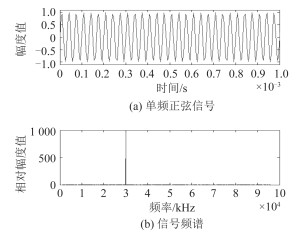图 2 频率为30 kHz的正弦信号时频图 Fig. 2 30 kHz Sine signal in time and frequency domain

NCO本振信号频率为24kHz，采样率为192kHz，产生正交的正、余弦分量，与输入信号混频，进行频谱搬移。图 3为正余弦分量信号时域波形，截取一定时间。图 4为混频后的输出信号。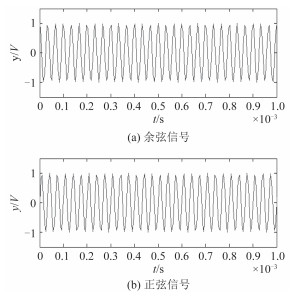图 3 本振信号24 kHz Fig. 3 24 kHz Sine and cosine signal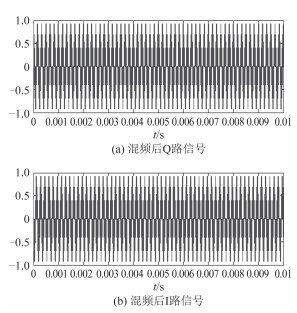图 4 混频后的I、Q时域信号 Fig. 4 I and Q singal after frequency mixing
2.2 FIR滤波器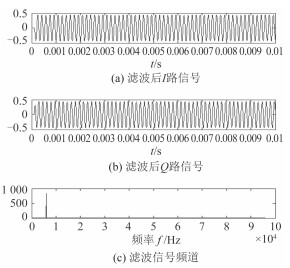图 5 FIR滤波后的时频图 Fig. 5 I and Q signal in time and frequency domain after FIR filter
2.3 数据抽取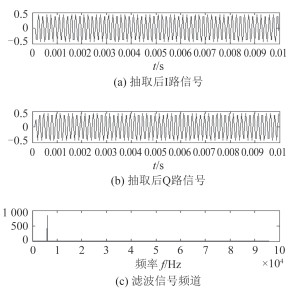图 6 数据抽取的时频信号图 Fig. 6 I and Q signal in time and frequency domain after data decimation
3 下变频的FPGA实现

FPGA芯片选择为Altera公司的CycloneIII的EP3C120型号，芯片内部具有119k的LE单元，288个18bit×18bit的乘法器，片内RAM有3.88kbits，系统的主时钟为50MHz，应用Verilog语言编程+IP核的设计的设计方法。

3.1 NCO模块的实现

NCO主要产生正交的正余弦分量，其实质是实现频率的合成，而直接数字频率合成（DDS）技术是一种全新的频率合成技术，具有频率分辨率高、转换速度快和相位连续等特点。在EP3C120的IP核库中，具有DDS模块，可以配置参数生成特定的输出信号。其模块如图 7所示。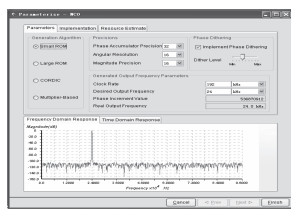图 7 DDS的配置参数示意图 Fig. 7 DDS configuration diagram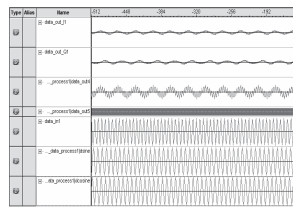图 8 Signaltap中DDS输出信号 Fig. 8 DDS outputs in signaltap II
3.2 混频器的实现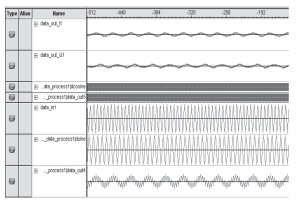图 9 混频后的signaltap中的信号量 Fig. 9 Signals in signaltap after multiplication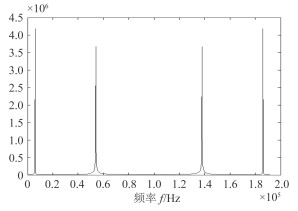图 10 混频后的频谱图 Fig. 10 The frequency spectrum after multiplication
3.3 FIR低通滤波的实现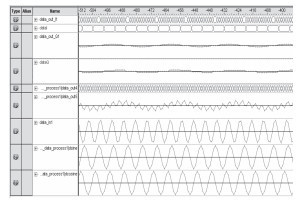图 11 滤波及数据抽取后的信号量 Fig. 11 Signals in signaltap after filter and decimation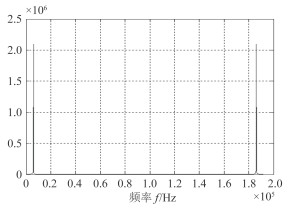图 12 滤波后的信号频谱图 Fig. 12 The frequency spectrum after filter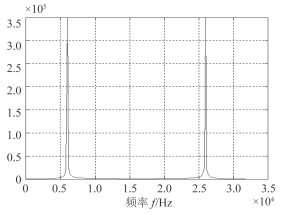图 13 数据抽取后的频谱图 Fig. 13 The frequency spectrum after data decimation
3.4 数据的抽取

4 结  语

  曹李秋.软件无线电数字下变频FPGA实现的研究[D].武汉:武汉理工大学, 2006. http://cdmd.cnki.com.cn/article/cdmd-10497-2006061306.htm  高亚军. 基于FPGA的数字信号处理[M]. 北京: 电子工业出版社, 2012 : 121 -130.  陈东坡.数字下变频电路的FPGA实现[D].西安:西安电子科技大学, 2009. http://cdmd.cnki.com.cn/article/cdmd-10701-2009195642.htm  周俊峰, 陈涛. 基于FPGA的直接数字频率合成器的设计和实现[J]. 电子技术应用 , 2002, 28 (8) :34–36.  胡跃, 张正鸿. 基于FPGA的数字正交混频变换算法的实现[J]. 国外电子测量技术 , 2007, 26 (5) :9–11.  奥本海默A V, 谢费R W, 巴克J R.离散时间信号处理[M].刘树棠, 黄建国, 译.西安:西安交通大学出版社, 2007: 352-412.  西瑞克斯(北京)通信设备有限公司. 无线通信的MATLAB和FPGA实现[M]. 北京: 人民邮电出版社, 2009 : 90 -97.  胡广书. 数字信号处理理论、算法与实现第3版[M]. 北京: 清华大学, 2012 : 355 -363.